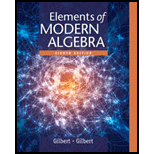# Evaluate each of the following sets, where U = { 0 , 1 , 2 , 3 , … , 10 } A = { 0 , 1 , 2 , 3 , 4 , 5 } B = { 0 , 2 , 4 , 6 , 8 , 10 } C = { 2 , 3 , 5 , 7 } . a. A ∪ B b. A ∩ C c. A ′ ∪ B d. A ∩ B ∩ C e. A ′ ∩ B ∩ C f. A ∪ ( B ∩ C ) g. A ∩ ( B ∪ C ) h. ( A ∪ B ′ ) ′ i. A − B j. B − A k. A − ( B − C ) l. C − ( B − A ) m. ( A − B ) ∩ ( C − B ) n. ( A − B ) ∩ ( A − C )### Elements Of Modern Algebra

8th Edition
Gilbert + 2 others
Publisher: Cengage Learning,
ISBN: 9781285463230### Elements Of Modern Algebra

8th Edition
Gilbert + 2 others
Publisher: Cengage Learning,
ISBN: 9781285463230

#### Solutions

Chapter
Section
Chapter 1.1, Problem 5E
Textbook Problem

## Expert Solution

### Want to see the full answer?

Check out a sample textbook solution.See solution

### Want to see this answer and more?

Experts are waiting 24/7 to provide step-by-step solutions in as fast as 30 minutes!*

See Solution

*Response times vary by subject and question complexity. Median response time is 34 minutes and may be longer for new subjects.